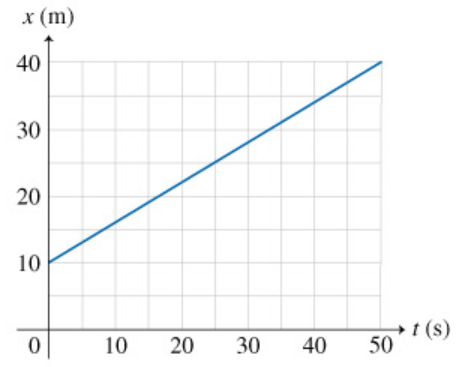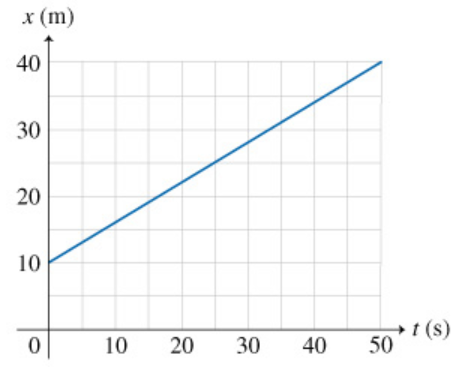# Problem: To describe the motion of a particle along a straight line, it is often convenient to draw a graph representing the position of the particle at different times. This type of graph is usually referred to as an x x vs. t t graph. To draw such a graph, choose an axis system in which time t t is plotted on the horizontal axis and position x x on the vertical axis. Then, indicate the values of x x at various times t t . Mathematically, this corresponds to plotting the variable x x as a function of t t . An example of a graph of position as a function of time for a particle traveling along a straight line is shown below. Note that an x x vs. t t graph like this does not represent the path of the particle in space.PART AWhat is the overall displacement ΔxΔx of the particle?PART BWhat is the average velocity vavvav of the particle over the time interval Δt=50.0sΔt=50.0s ?Express your answer in meters per second.PART CWhat is the instantaneous velocity vv of the particle at t=10.0st=10.0s?Express your answer in meters per second.Another common graphical representation of motion along a straight line is the vv vs. tt, that is, the graph of (instantaneous) velocity as a function of time. In this graph, time tt is plotted on the horizontal axis and velocity vv on the vertical axis. Note that by definition, velocity and acceleration are vector quantities. In straight-line motion, however, these vectors have only one nonzero component in the direction of motion. Thus, in this problem, we will call vv aa the acceleration, even though they are really the components of the velocity and acceleration vectors in the direction of motion.PART DWhich of the graphs shown is the correct vv vs. tt plot for the motion described in the previous parts?

###### FREE Expert Solution

Part A

The overall displacement:

ΔxΔx = xf - xi

ΔxΔx = 40 - 10 = 30 m

The overall displacement ΔxΔx of the particle is 30 m.

100% (473 ratings)###### Problem Details

To describe the motion of a particle along a straight line, it is often convenient to draw a graph representing the position of the particle at different times. This type of graph is usually referred to as an x x vs. t t graph. To draw such a graph, choose an axis system in which time t t is plotted on the horizontal axis and position x x on the vertical axis. Then, indicate the values of x x at various times t t . Mathematically, this corresponds to plotting the variable x x as a function of t t . An example of a graph of position as a function of time for a particle traveling along a straight line is shown below. Note that an x x vs. t t graph like this does not represent the path of the particle in space.PART A

What is the overall displacement ΔxΔx of the particle?

PART B

What is the average velocity vavvav of the particle over the time interval Δt=50.0sΔt=50.0s ?

PART C

What is the instantaneous velocity vv of the particle at t=10.0st=10.0s?

Another common graphical representation of motion along a straight line is the vv vs. tt, that is, the graph of (instantaneous) velocity as a function of time. In this graph, time tt is plotted on the horizontal axis and velocity vv on the vertical axis. Note that by definition, velocity and acceleration are vector quantities. In straight-line motion, however, these vectors have only one nonzero component in the direction of motion. Thus, in this problem, we will call vv aa the acceleration, even though they are really the components of the velocity and acceleration vectors in the direction of motion.

PART D

Which of the graphs shown is the correct vv vs. tt plot for the motion described in the previous parts?